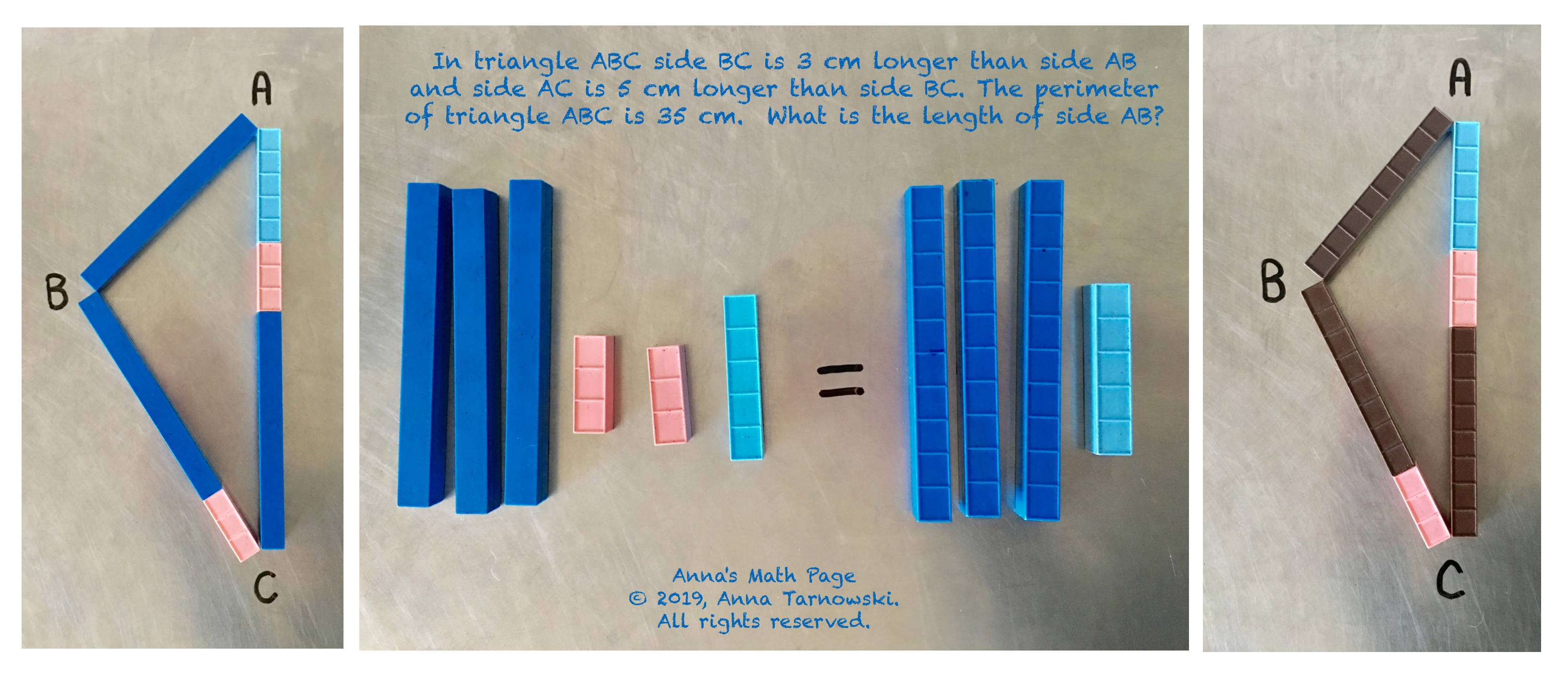# Find Expressions and Equation and Solve for Side Lengths of TriangleOther than labelling the triangle and using the same as sign there were no other symbols needed to solve this problem. The models show the expressions c, c+3, and c+3+5 and the equation c+c+3+c+3+5=35 or 3c+11=35. Using same as, hero zero, and no fun get back to one children can see that c=8.